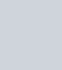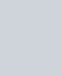• 欢迎访问图书库 网站，为您分享各种电子书，让我们有学习的平台。

# `《指数基金投资从入门到精通》电子书`

``` 经济管理 adminlele 1年前 (2022-05-13) 120次浏览 已收录 0个评论 扫描二维码 ```

``

` (adsbygoogle=window.adsbygoogle||[]).push({}) `

## `PDF电子书内容`

`本书的写作初衷是写一本让大家能看懂的、干货满满的、注重实战而不是理论知识和教条堆砌的指数投资书籍。对于很多上班族而言，如果平常几乎没有研究股票和基金的时间和精力，那么定投指数基金将会是一个不错的投资方式，这类投资者可以好好看看老罗关于定投章节的内容，从中可以学习到定投的优势、定投金额、定投品种以及止盈的方式等相关知识。`

` (adsbygoogle=window.adsbygoogle||[]).push({})`

`对于爱好学习以及时间充裕的投资者来说，可以通过学习本书，掌握如何挑选一只好的指数基金，学会运用指数估值数据选择指数基金，并且在不同市场环境下合理运用不同策略投资指数基金，了解指数基金产品进行低风险套利的方法，最终通过学会更多的指数投资相关知识，来帮助自己更好地进行投资理财。`

`本书的写法由浅入深，既适合基金投资的新手，也适合希望进一步提升指数投资能力的投资老鸟。在每个章节的最后，老罗都会谈谈自己对该节内容的感悟和理解，帮助大家更好地理解每个章节的内容。`

## `PDF电子书作者信息`

`老罗，广发基金公司指数投资部负责人兼基金经理、雪球大V（ID：老罗话指数投资），江湖人称老罗，多年来专注指数及量化投资领域。曾多次在包含证券三大报在内的财经媒体发表指数投资相关的文章，并长期担任沪深交易所“指数基金及ETF特约讲师”。`

`秉承“授人以鱼不如授人以渔”的原则，老罗结合自己多年的指数投资一线从业经历，以原创为主的方式，向广大投资者传授“指数及指数基金投资理念、投资技巧”。其运营的“老罗话指数投资”雪球专栏（ID：老罗话指数投资）和微信公众号（ID：index_fund）订阅用户已超过10万，成为颇受投资者欢迎的指数投资自媒体号。`

` (adsbygoogle=window.adsbygoogle||[]).push({})`

`对于投资经验不足的中小投资者，老罗更推崇以指数基金定投的方式介入市场，并在其公众号中详细阐述了定投的理念及投资方法。投资是贯穿投资者一生的长期事业，老罗深知“独行者速，众行者远”。因此，愿意以开放的心态将自己在指数投资管理过程中的所思所想与投资者共同分享，也达到鞭策自己的作用。`

`同时，作为一名指数与量化行业的从业者，老罗更偏向用“数据说话”，所发布的文章一般除要求有逻辑外，还要求有数据支持，包括公众号中每个交易日发布的市场主流指数估值及历史分位等数据，相信都可以成为投资者投资指数基金的重要参考。老罗真心希望“老罗话指数投资”这一平台能够为投资者带来实实在在的能力提升，也希望投资者与老罗共同努力，我们一起在投资的征途上走得更远。`

## `PDF电子书章节目录`

`第1章 指数基金的魅力`

`第2章 指数的介绍`

` (adsbygoogle=window.adsbygoogle||[]).push({})`

`第3章 指数基金投资入门知识`

`第4章 指数基金技巧`

`第5章 ETF基金`

`第6章 分级基金`

`第7章 基金定投入门篇`

`第8章 基金定投高级篇`

`第9章 指数基金投资策略篇`

`指数基金投资从入门到精通电子书下载，这是一本教你怎么玩指数投资的书籍，无论你是新手还是老手都可以通过这本书籍来获得知识和投资指导，值得一看。`

## `下载地址：`

`zsjjtzrmjt.zip: http://xz.tbookk.com/f/560517-579431095-f81b52?p=311929`

`此处内容已经被作者隐藏，请输入验证码查看内容验证码：请关注本站微信公众号，回复“验证码”，获取验证码。在微信里搜索“杰哥读书”或者“tbookk”或者微信扫描右侧二维码都可以关注本站微信公众号。`

```更多万册书：http://dd.ma/ZOcgL2Yr 请通过以下商城购买正版书籍图书库 , 版权所有丨如未注明 , 均为原创丨本网站采用BY-NC-SA协议进行授权 转载请注明原文链接：《指数基金投资从入门到精通》电子书本站电子书版权归原作者及开发商所有，仅限个人测试交流学习之用，请在下载后24小时内删除。若有违反您个人权益，请留言反馈删除相关信息。```
``` 喜欢 (0)赏[感谢您的支持与关注！]分享 (0)```
``` !function(t,e){"object"==typeof exports&&"undefined"!=typeof module?module.exports=e():"function"==typeof define&&define.amd?define(e):(t="undefined"!=typeof globalThis?globalThis:t||self).LazyLoad=e()}(this,function(){"use strict";function e(){return(e=Object.assign||function(t){for(var e=1;e<arguments.length;e++){var n,a=arguments[e];for(n in a)Object.prototype.hasOwnProperty.call(a,n)&&(t[n]=a[n])}return t}).apply(this,arguments)}function i(t){return e({},it,t)}function o(t,e){var n,a="LazyLoad::Initialized",i=new t(e);try{n=new CustomEvent(a,{detail:{instance:i}})}catch(t){(n=document.createEvent("CustomEvent")).initCustomEvent(a,!1,!1,{instance:i})}window.dispatchEvent(n)}function l(t,e){return t.getAttribute(gt+e)}function c(t){return l(t,bt)}function s(t,e){return function(t,e,n){e=gt+e;null!==n?t.setAttribute(e,n):t.removeAttribute(e)}(t,bt,e)}function r(t){return s(t,null),0}function u(t){return null===c(t)}function d(t){return c(t)===vt}function f(t,e,n,a){t&&(void 0===a?void 0===n?t(e):t(e,n):t(e,n,a))}function _(t,e){nt?t.classList.add(e):t.className+=(t.className?" ":"")+e}function v(t,e){nt?t.classList.remove(e):t.className=t.className.replace(new RegExp("(^|\\s+)"+e+"(\\s+|\$)")," ").replace(/^\s+/,"").replace(/\s+\$/,"")}function g(t){return t.llTempImage}function b(t,e){!e||(e=e._observer)&&e.unobserve(t)}function p(t,e){t&&(t.loadingCount+=e)}function h(t,e){t&&(t.toLoadCount=e)}function n(t){for(var e,n=[],a=0;e=t.children[a];a+=1)"SOURCE"===e.tagName&&n.push(e);return n}function m(t,e){(t=t.parentNode)&&"PICTURE"===t.tagName&&n(t).forEach(e)}function a(t,e){n(t).forEach(e)}function E(t){return!!t[st]}function I(t){return t[st]}function y(t){return delete t[st]}function A(e,t){var n;E(e)||(n={},t.forEach(function(t){n[t]=e.getAttribute(t)}),e[st]=n)}function k(a,t){var i;E(a)&&(i=I(a),t.forEach(function(t){var e,n;e=a,(t=i[n=t])?e.setAttribute(n,t):e.removeAttribute(n)}))}function L(t,e,n){_(t,e.class_loading),s(t,ut),n&&(p(n,1),f(e.callback_loading,t,n))}function w(t,e,n){n&&t.setAttribute(e,n)}function x(t,e){w(t,ct,l(t,e.data_sizes)),w(t,rt,l(t,e.data_srcset)),w(t,ot,l(t,e.data_src))}function O(t,e,n){var a=l(t,e.data_bg_multi),i=l(t,e.data_bg_multi_hidpi);(a=at&&i?i:a)&&(t.style.backgroundImage=a,n=n,_(t=t,(e=e).class_applied),s(t,ft),n&&(e.unobserve_completed&&b(t,e),f(e.callback_applied,t,n)))}function N(t,e){!e||0<e.loadingCount||0<e.toLoadCount||f(t.callback_finish,e)}function C(t,e,n){t.addEventListener(e,n),t.llEvLisnrs[e]=n}function M(t){return!!t.llEvLisnrs}function z(t){if(M(t)){var e,n,a=t.llEvLisnrs;for(e in a){var i=a[e];n=e,i=i,t.removeEventListener(n,i)}delete t.llEvLisnrs}}function R(t,e,n){var a;delete t.llTempImage,p(n,-1),(a=n)&&--a.toLoadCount,v(t,e.class_loading),e.unobserve_completed&&b(t,n)}function T(o,r,c){var l=g(o)||o;M(l)||function(t,e,n){M(t)||(t.llEvLisnrs={});var a="VIDEO"===t.tagName?"loadeddata":"load";C(t,a,e),C(t,"error",n)}(l,function(t){var e,n,a,i;n=r,a=c,i=d(e=o),R(e,n,a),_(e,n.class_loaded),s(e,dt),f(n.callback_loaded,e,a),i||N(n,a),z(l)},function(t){var e,n,a,i;n=r,a=c,i=d(e=o),R(e,n,a),_(e,n.class_error),s(e,_t),f(n.callback_error,e,a),i||N(n,a),z(l)})}function G(t,e,n){var a,i,o,r,c;t.llTempImage=document.createElement("IMG"),T(t,e,n),E(c=t)||(c[st]={backgroundImage:c.style.backgroundImage}),o=n,r=l(a=t,(i=e).data_bg),c=l(a,i.data_bg_hidpi),(r=at&&c?c:r)&&(a.style.backgroundImage='url("'.concat(r,'")'),g(a).setAttribute(ot,r),L(a,i,o)),O(t,e,n)}function D(t,e,n){var a;T(t,e,n),a=e,e=n,(t=It[(n=t).tagName])&&(t(n,a),L(n,a,e))}function V(t,e,n){var a;a=t,(-1<yt.indexOf(a.tagName)?D:G)(t,e,n)}function F(t,e,n){var a;t.setAttribute("loading","lazy"),T(t,e,n),a=e,(e=It[(n=t).tagName])&&e(n,a),s(t,vt)}function j(t){t.removeAttribute(ot),t.removeAttribute(rt),t.removeAttribute(ct)}function P(t){m(t,function(t){k(t,Et)}),k(t,Et)}function S(t){var e;(e=At[t.tagName])?e(t):E(e=t)&&(t=I(e),e.style.backgroundImage=t.backgroundImage)}function U(t,e){var n;S(t),n=e,u(e=t)||d(e)||(v(e,n.class_entered),v(e,n.class_exited),v(e,n.class_applied),v(e,n.class_loading),v(e,n.class_loaded),v(e,n.class_error)),r(t),y(t)}function \$(t,e,n,a){var i;n.cancel_on_exit&&(c(t)!==ut||"IMG"===t.tagName&&(z(t),m(i=t,function(t){j(t)}),j(i),P(t),v(t,n.class_loading),p(a,-1),r(t),f(n.callback_cancel,t,e,a)))}function q(t,e,n,a){var i,o,r=(o=t,0<=pt.indexOf(c(o)));s(t,"entered"),_(t,n.class_entered),v(t,n.class_exited),i=t,o=a,n.unobserve_entered&&b(i,o),f(n.callback_enter,t,e,a),r||V(t,n,a)}function H(t){return t.use_native&&"loading"in HTMLImageElement.prototype}function B(t,i,o){t.forEach(function(t){return(a=t).isIntersecting||0<a.intersectionRatio?q(t.target,t,i,o):(e=t.target,n=t,a=i,t=o,void(u(e)||(_(e,a.class_exited),\$(e,n,a,t),f(a.callback_exit,e,n,t))));var e,n,a})}function J(e,n){var t;et&&!H(e)&&(n._observer=new IntersectionObserver(function(t){B(t,e,n)},{root:(t=e).container===document?null:t.container,rootMargin:t.thresholds||t.threshold+"px"}))}function K(t){return Array.prototype.slice.call(t)}function Q(t){return t.container.querySelectorAll(t.elements_selector)}function W(t){return c(t)===_t}function X(t,e){return e=t||Q(e),K(e).filter(u)}function Y(e,t){var n;(n=Q(e),K(n).filter(W)).forEach(function(t){v(t,e.class_error),r(t)}),t.update()}function t(t,e){var n,a,t=i(t);this._settings=t,this.loadingCount=0,J(t,this),n=t,a=this,Z&&window.addEventListener("online",function(){Y(n,a)}),this.update(e)}var Z="undefined"!=typeof window,tt=Z&&!("onscroll"in window)||"undefined"!=typeof navigator&&/(gle|ing|ro)bot|crawl|spider/i.test(navigator.userAgent),et=Z&&"IntersectionObserver"in window,nt=Z&&"classList"in document.createElement("p"),at=Z&&1<window.devicePixelRatio,it={elements_selector:".lazy",container:tt||Z?document:null,threshold:300,thresholds:null,data_src:"src",data_srcset:"srcset",data_sizes:"sizes",data_bg:"bg",data_bg_hidpi:"bg-hidpi",data_bg_multi:"bg-multi",data_bg_multi_hidpi:"bg-multi-hidpi",data_poster:"poster",class_applied:"applied",class_loading:"litespeed-loading",class_loaded:"litespeed-loaded",class_error:"error",class_entered:"entered",class_exited:"exited",unobserve_completed:!0,unobserve_entered:!1,cancel_on_exit:!0,callback_enter:null,callback_exit:null,callback_applied:null,callback_loading:null,callback_loaded:null,callback_error:null,callback_finish:null,callback_cancel:null,use_native:!1},ot="src",rt="srcset",ct="sizes",lt="poster",st="llOriginalAttrs",ut="loading",dt="loaded",ft="applied",_t="error",vt="native",gt="data-",bt="ll-status",pt=[ut,dt,ft,_t],ht=[ot],mt=[ot,lt],Et=[ot,rt,ct],It={IMG:function(t,e){m(t,function(t){A(t,Et),x(t,e)}),A(t,Et),x(t,e)},IFRAME:function(t,e){A(t,ht),w(t,ot,l(t,e.data_src))},VIDEO:function(t,e){a(t,function(t){A(t,ht),w(t,ot,l(t,e.data_src))}),A(t,mt),w(t,lt,l(t,e.data_poster)),w(t,ot,l(t,e.data_src)),t.load()}},yt=["IMG","IFRAME","VIDEO"],At={IMG:P,IFRAME:function(t){k(t,ht)},VIDEO:function(t){a(t,function(t){k(t,ht)}),k(t,mt),t.load()}},kt=["IMG","IFRAME","VIDEO"];return t.prototype={update:function(t){var e,n,a,i=this._settings,o=X(t,i);{if(h(this,o.length),!tt&&et)return H(i)?(e=i,n=this,o.forEach(function(t){-1!==kt.indexOf(t.tagName)&&F(t,e,n)}),void h(n,0)):(t=this._observer,i=o,t.disconnect(),a=t,void i.forEach(function(t){a.observe(t)}));this.loadAll(o)}},destroy:function(){this._observer&&this._observer.disconnect(),Q(this._settings).forEach(function(t){y(t)}),delete this._observer,delete this._settings,delete this.loadingCount,delete this.toLoadCount},loadAll:function(t){var e=this,n=this._settings;X(t,n).forEach(function(t){b(t,e),V(t,n,e)})},restoreAll:function(){var e=this._settings;Q(e).forEach(function(t){U(t,e)})}},t.load=function(t,e){e=i(e);V(t,e)},t.resetStatus=function(t){r(t)},Z&&function(t,e){if(e)if(e.length)for(var n,a=0;n=e[a];a+=1)o(t,n);else o(t,e)}(t,window.lazyLoadOptions),t});!function(e,t){"use strict";function a(){t.body.classList.add("litespeed_lazyloaded")}function n(){console.log("[LiteSpeed] Start Lazy Load Images"),d=new LazyLoad({elements_selector:"[data-lazyloaded]",callback_finish:a}),o=function(){d.update()},e.MutationObserver&&new MutationObserver(o).observe(t.documentElement,{childList:!0,subtree:!0,attributes:!0})}var d,o;e.addEventListener?e.addEventListener("load",n,!1):e.attachEvent("onload",n)}(window,document);var litespeed_vary=document.cookie.replace(/(?:(?:^|.*;\s*)_lscache_vary\s*\=\s*([^;]*).*\$)|^.*\$/,"");litespeed_vary||fetch("/wp-content/plugins/litespeed-cache/guest.vary.php",{method:"POST",cache:"no-cache",redirect:"follow"}).then(e=>e.json()).then(e=>{console.log(e),e.hasOwnProperty("reload")&&"yes"==e.reload&&(sessionStorage.setItem("litespeed_docref",document.referrer),window.location.reload(!0))});const litespeed_ui_events=["mouseover","click","keydown","wheel","touchmove","touchstart"];var urlCreator=window.URL||window.webkitURL;function litespeed_load_delayed_js_force(){console.log("[LiteSpeed] Start Load JS Delayed"),litespeed_ui_events.forEach(e=>{window.removeEventListener(e,litespeed_load_delayed_js_force,{passive:!0})}),document.querySelectorAll("iframe[data-litespeed-src]").forEach(e=>{e.setAttribute("src",e.getAttribute("data-litespeed-src"))}),"loading"==document.readyState?window.addEventListener("DOMContentLoaded",litespeed_load_delayed_js):litespeed_load_delayed_js()}litespeed_ui_events.forEach(e=>{window.addEventListener(e,litespeed_load_delayed_js_force,{passive:!0})});async function litespeed_load_delayed_js(){let t=[];for(var d in document.querySelectorAll('script[type="litespeed/javascript"]').forEach(e=>{t.push(e)}),t)await new Promise(e=>litespeed_load_one(t[d],e));document.dispatchEvent(new Event("DOMContentLiteSpeedLoaded")),window.dispatchEvent(new Event("DOMContentLiteSpeedLoaded"))}function litespeed_load_one(t,e){console.log("[LiteSpeed] Load ",t);var d=document.createElement("script");d.addEventListener("load",e),d.addEventListener("error",e),t.getAttributeNames().forEach(e=>{"type"!=e&&d.setAttribute("data-src"==e?"src":e,t.getAttribute(e))});let a=!(d.type="text/javascript");!d.src&&t.textContent&&(d.src=litespeed_inline2src(t.textContent),a=!0),t.after(d),t.remove(),a&&e()}function litespeed_inline2src(t){try{var d=urlCreator.createObjectURL(new Blob([t.replace(/^(?:<!--)?(.*?)(?:-->)?\$/gm,"\$1")],{type:"text/javascript"}))}catch(e){d="data:text/javascript;base64,"+btoa(t.replace(/^(?:<!--)?(.*?)(?:-->)?\$/gm,"\$1"))}return d} ```